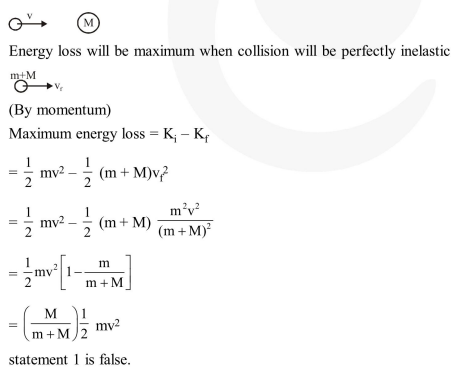Deepak Scored 45->99%ile with Bounce Back Crack Course. You can do it too!

# This question has Statement

Question:

This question has Statement I and Statement II. Of the four choices given after the Statements, choose the one that best describes the two Statements.

Statement - I : A point particle of mass m moving with speed v collides with stationary point particle of mass M. If the maximum energy loss possible is given as $f\left(\frac{1}{2} m v^{2}\right)$ then $f=\left(\frac{m}{M+m}\right)$.

Statement - II : Maximum energy loss occurs when the particles get stuck together as a result of the collision.

1. Statement-I is true, Statement-II is true, Statement-II is a correct explanation of Statement-I.

2. Statement-I is true, Statement-II is true, Statement-II is a not correct explanation of Statement-I.

3. Statement-I is true, Statement-II is false.

4. Statement-I is false, Statement-II is true

Correct Option: , 4

Solution: